# IBPS RRB 2018 Prelims : Quants – Day 8

Dear Bankersdaily Aspirants,

IBPS RRB PO & Office Assistant 2018 Preliminary Examination starts from 11th August, 2018. The IBPS RRB Exam 2018 is one of the most expected exam of the year. The reason for the consummate expectation is different from that of the others.

The number of candidates attending the IBPS RRB PO & Office Assistant is high when compared to the other exams like SBI & Insurance Exams. The other important factor is that , no English section is there in the preliminary examination of the IBPS RRB PO & Assistant Exams. This is really a boost to the candidates as many of the aspirants couldn’t get the requisite marks in the English section and this exam is the best bet for the aspirants.

Since only few days are left for the IBPS RRB PO & Assistant Exam 2018 Preliminary Exam , we will be providing daily 20 Questions in Quantitative Aptitude & Reasoning Section to evoke the preparation mode.

Aspirants can answer the Questions by commenting the answers for the Questions in the comments section. We will be providing the answers for the Questions @ 6:00 P.M everyday.

## IBPS RRB PO & Assistant 2018 – Prelims Exam – Day 8

Topic : Quantitative Aptitude

Total Number of Questions : 20

Total Time : 15 minutes

D.1-5) What value should come in place of question mark (?) in the following equations?

Q.1)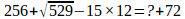a) 34

b) 56

c) 72

d) 48

e) 27

Q.2)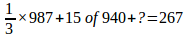a) 203

b) 213

c) -203

d) -222

e) -237

Q.3)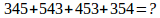a) 1659

b) 1695

c) 1879

d) 1873

e) 1768

Q.4)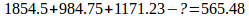a) 3545

b) 3354

c) 3544

d) 3445

e) 3454

Q.5)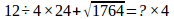a) 25.5

b) 32.4

c) 28.5

d) 29.7

e) 43.6

Q.6) In a family of four members, the average height of a family is 173 cm. If a person is added the family, then the average is decreased by 2cm. What is the height of the new person join in the family?

a) 158 cm

b) 166 cm

c) 155 cm

d) 172 cm

e) 163 cm

Q.7) A, B and C can do a piece of work in 8 days, 12 days and 16 days respectively. A and B work for three days and how many days will it take for only C to complete the remaining work?

a) 6 days

b) 11 days

c) 10 days

d) 13 days

e) 14 days

Q.8) How many kg of sugar at 45 paise per kg must a man mix with 20 kg of sugar at 23 paise per kg. So that he may on selling the mixture at 32 paise per kg, gain 28% on the outlay?

a) 4 kg

b) 8 kg

c) 3 kg

d) 2 kg

e) 6 kg

Q.9) A trader sells two bikes for Rs. 27025 each neither losing nor gaining in total. If he sold one of the bikes at a gain of 15% and the other is sold at a loss of how many percentage? (Approximately)

a) 8.7%

b) 12.4%

c) 11.5%

d) 10.9%

e) 15.3%

Q.10) A man purchased a table and chair. The chair costs 10% more than the table. The cost price of a table and a chair together is Rs. 8000. Find the cost price of a table? (Approximately)

a) Rs. 3480

b) Rs. 3780

c) Rs. 3810

d) Rs. 3240

e) Rs. 3980

D.11-15) In the below questions, two equations I and II are given. You have to solve both the equations and answer the following questions accordingly.

Q.11)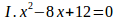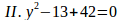a) X>Y

b) Y>X

c) X=Y or Relationship can’t be established between x and y.

d) X≤Y

e) X≥Y

Q.12)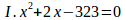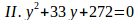a) X>Y

b) Y>X

c) X=Y or Relationship can’t be established between x and y.

d) X≤Y

e) X≥Y

Q.13)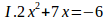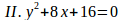a) X>Y

b) Y>X

c) X=Y or Relationship can’t be established between x and y.

d) X≤Y

e) X≥Y

Q.14)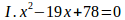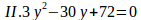a) X>Y

b) Y>X

c) X=Y or Relationship can’t be established between x and y.

d) X≤Y

e) X≥Y

Q.15)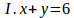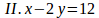a) X>Y

b) Y>X

c) X=Y or Relationship can’t be established between x and y.

d) X≤Y

e) X≥Y

D.16-20) Find the wrong number and answers the following questions.

Q.16) 45 117 201 301 426 592 826

a) 45

b) 826

c) 601

d) 426

e) 117

Q.17) 52, 59, 194, 976, 6860, 61775

a) 976

b) 6860

c) 59

d) 61775

e) 194

Q.18) 358, 390, 322, 458, 186, 730

a) 358

b) 390

c) 322

d) 458

e) 730

Q.19) 453, 1182, 2125, 3513, 5241, 7438

a) 1182

b) 2125

c) 3513

d) 5241

e) 453

Q.20) 6, 11, 52, 480, 7696, 192420

a) 6

b) 52

c) 7696

d) 11

e) 480

Explanations

Q.1) e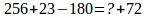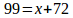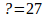Q.2) c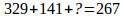?=-203

Q.3) b?=1695

Q.4) d?=3445

Q.5) c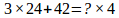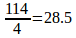Q.6) e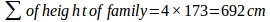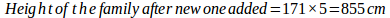Height of the new person=855-692= 163 cm

Q.7) a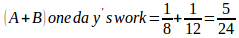A+B’s three days work=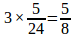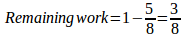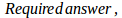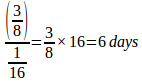Q.8) d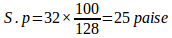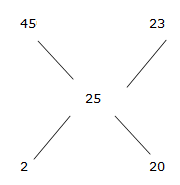Ratio=1 : 10

23 paise per kg sugar=10x

10x=20

x=2 kg

45 paisa per kg sugar= x

=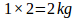Q.9) c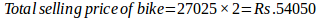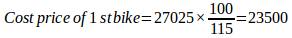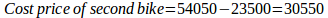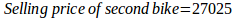Loss=30550-27025=Rs.3525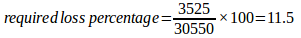Q.10) c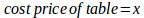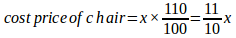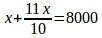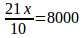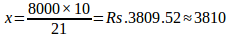Q.11) d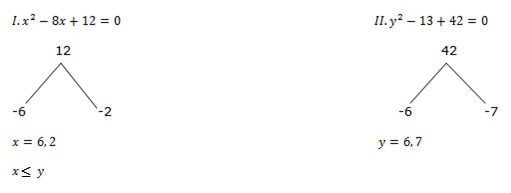Q.12) c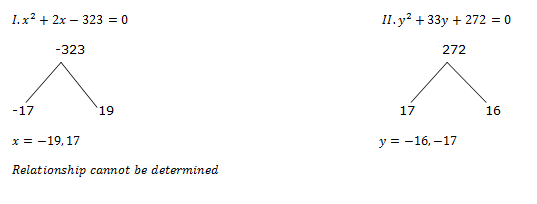Q.13) a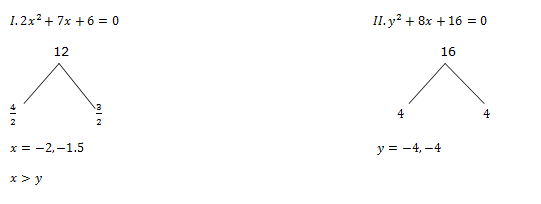Q.14) e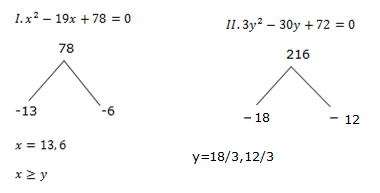Q.15) a(I)(II)

Multiply by 2 in equation I,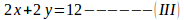Solve the equation II and III, and we get,

x=8 and y=-2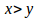Q.16) b

The difference is,

72      84    100   125   166     232

12     16      25     41     66

4        9       16     25

Hence, 826 is the wrong number.

Q.17) e

The series is,

52×1+7 = 59

59×3+14 = 191

191×5+21 = 976

976×7+28 = 6860

6860×9+35 = 61775

Hence, 194 is the wrong number.

Q.18) a

The difference is,

+34, -68, +136, -272, +544.

Hence, 358 is the wrong number.

Q.19) b

The difference is,

729, 1000, 1331, 1728, 2197

Hence, 2125 is the wrong number.

Q.20) a

The pattern is,

7×12+4 = 11

11×22+8 = 52

52×32+12 = 480

480×42+16 = 7696

7696×52+20 = 192420

Hence, 6 is the wrong number.

We will post this Practice Questions – Daily till the start of the IBPS RRB PO & Assistant (Regularly)

Quantitative Aptitude @ 3:00 P.M

Reasoning Ability -@ 5:00 P.M

 QUANTITATIVE APTITUDE REASONING DAY 1 DAY 1 DAY 2 DAY 2 DAY 3 DAY 3 DAY 4 DAY 4 DAY 5 DAY 5 DAY 6 DAY 6 DAY 7 DAY 7

Attend GEEK TEST – SOLVE 10 Daily and enhance your preparation to the next level. Attend the Daily Tests with the Timer and improve your solving ability.

Also Please attend this BLUE WHALE QUESTION (which is a combination of many topics and combined into a single one). This is a One Stop Solution for all your Miscellaneous needs.

## BLUE WHALE CHALLENGE QUESTIONS

Get the FULL LENGTH MOCK TEST SERIES for SBI PO Mains 2018 , SBI CLERK Mains 2018 & BOB PO Mains 2018 from our Bankersdaily Store.